# GSEB Solutions Class 12 Maths Chapter 3 Matrices Ex 3.3

Gujarat Board GSEB Textbook Solutions Class 12 Maths Chapter 3 Matrices Ex 3.3 Textbook Questions and Answers.

## Gujarat Board Textbook Solutions Class 12 Maths Chapter 3 Matrices Ex 3.3Question 1.
Find the transpose of each of the following matrices:
(i) $$\left[\begin{array}{c} 5 \\ 1 \\ \hline 2 \\ -1 \end{array}\right]$$
(ii) $$\left[\begin{array}{cc} 1 & -1 \\ 2 & 3 \end{array}\right]$$
(iii) $$\left[\begin{array}{ccc} -1 & 5 & 6 \\ \sqrt{3} & 5 & 6 \\ 2 & 3 & -1 \end{array}\right]$$
Solution: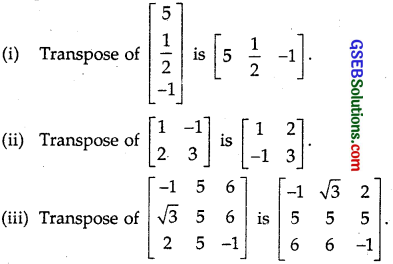Question 2.
If A = $$\left[\begin{array}{ccc} -1 & 2 & 3 \\ 5 & 7 & 9 \\ -2 & 1 & 1 \end{array}\right]$$ + B = $$\left[\begin{array}{ccc} -4 & 1 & -5 \\ 1 & 2 & 0 \\ 1 & 3 & 1 \end{array}\right]$$, then verify that
(i) (A+B)’ = A’ + B’
(ii) (A-B)’ = A’ – B’
Solution: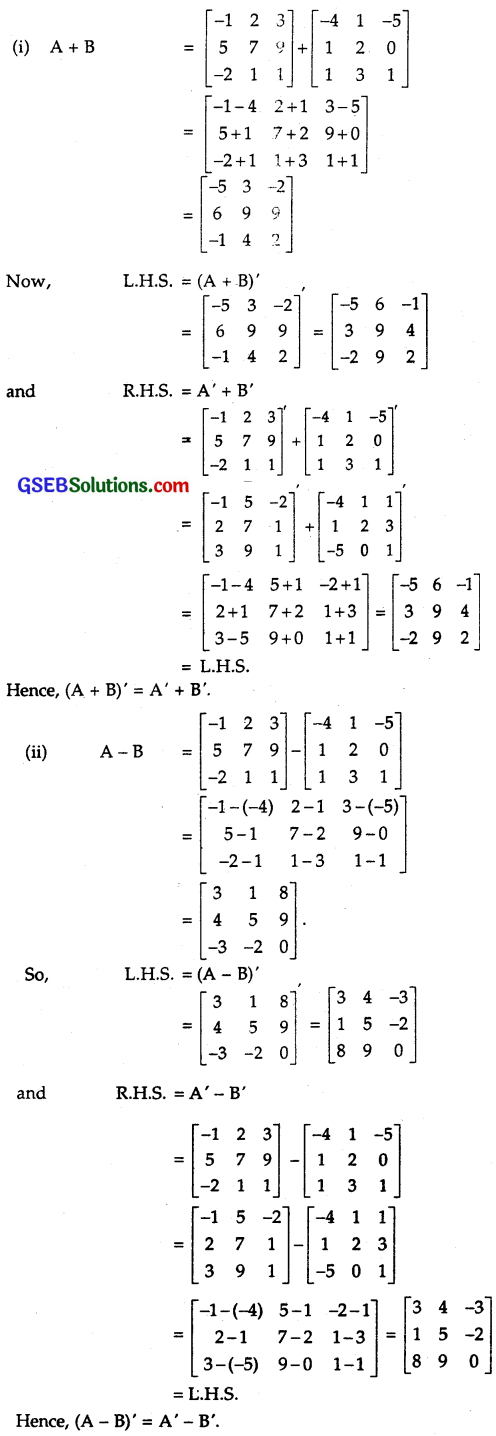Question 3.
If A’ = $$\left[\begin{array}{cc} 3 & 4 \\ -1 & 2 \\ 0 & 1 \end{array}\right]$$ + B = $$\left[\begin{array}{ccc} -1 & 2 & 1 \\ 1 & 2 & 3 \end{array}\right]$$, then verify that
(i) (A+B)’ = A’ + B’
(ii) (A-B)’ = A’ – B’
Solution: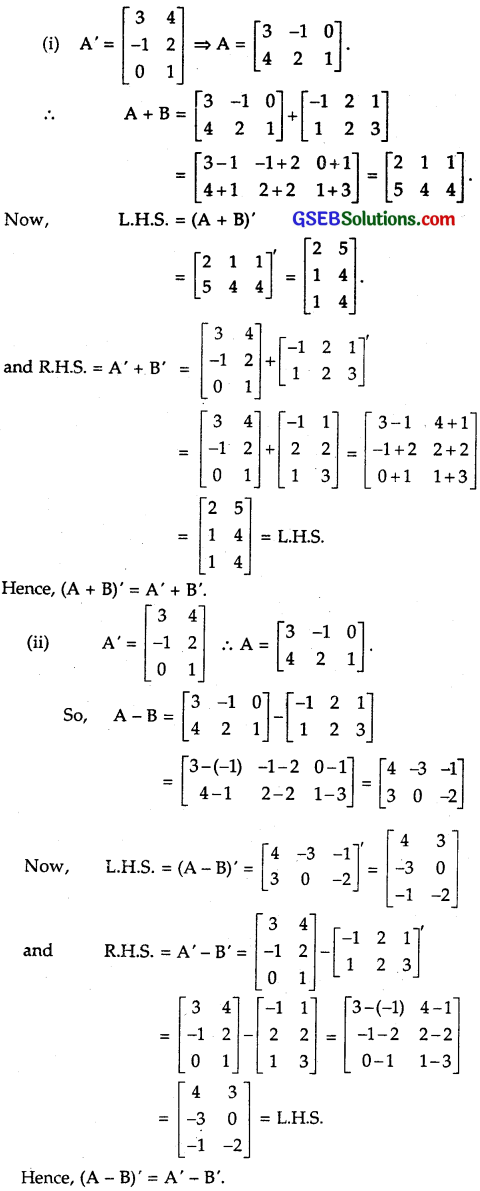Question 4.
If A’ = $$\left[\begin{array}{cc} -2 & 3 \\ 1 & 2 \end{array}\right]$$ and B = $$\left[\begin{array}{cc} -1 & 0 \\ 1 & 2 \end{array}\right]$$, then find (A + 2B’)
Solution:
A’ = $$\left[\begin{array}{cc} -2 & 3 \\ 1 & 2 \end{array}\right]$$. ∴ A = $$\left[\begin{array}{cc} -2 & 1 \\ 3 & 2 \end{array}\right]$$ and B = $$\left[\begin{array}{cc} -1 & 0 \\ 1 & 2 \end{array}\right]$$.
∴ A + 2B = $$\left[\begin{array}{cc} -2 & 1 \\ 3 & 2 \end{array}\right]$$ + 2$$\left[\begin{array}{cc} -1 & 0 \\ 1 & 2 \end{array}\right]$$
= $$\left[\begin{array}{cc} -2 & 1 \\ 3 & 2 \end{array}\right]$$ + $$\left[\begin{array}{cc} -2 & 0 \\ 2 & 4 \end{array}\right]$$ = $$\left[\begin{array}{cc} -2-2 & 1+0\\ 3+2 & 2+4 \end{array}\right]$$
= $$\left[\begin{array}{cc} -4 & 1 \\ 5 & 6 \end{array}\right]$$
∴(A + 2B)’ = $$\left[\begin{array}{cc} -4 & 1 \\ 5 & 6 \end{array}\right]$$ = $$\left[\begin{array}{cc} -4 & 5 \\ 1 & 6 \end{array}\right]$$.Question 5.
(i) A = $$\left[\begin{array}{c} 1 \\ -4 \\ 3 \end{array}\right]$$, B = $$\left[\begin{array}{lll} -1 & 2 & 1 \end{array}\right]$$
(ii) A = $$\left[\begin{array}{c} 0 \\ 1 \\ 2 \end{array}\right]$$, B = $$\left[\begin{array}{lll} 1 & 5 & 7 \end{array}\right]$$
Solution: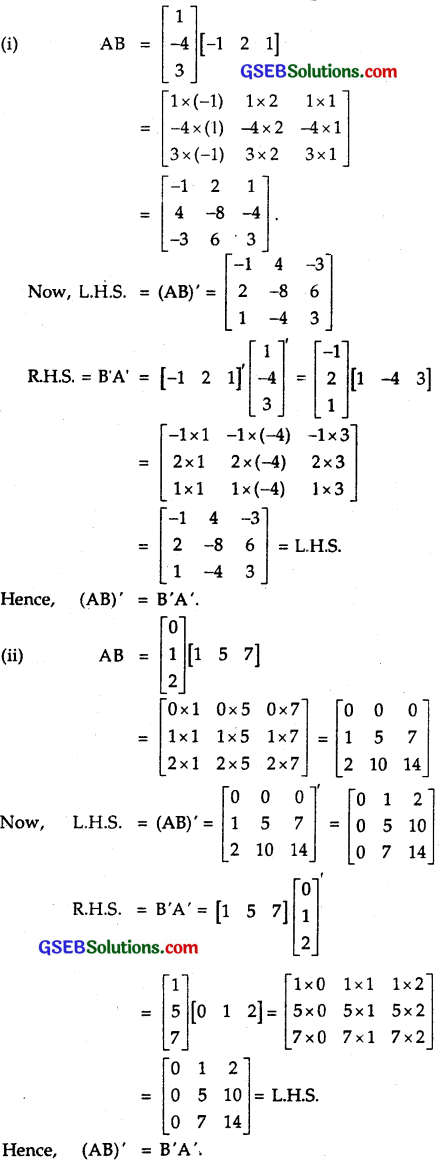Question 6.
If (i) A = $$\left[\begin{array}{cc} \cos \alpha & \sin \alpha \\ -\sin \alpha & \cos \alpha \end{array}\right]$$, then verify that A’A = I.

(ii) $$\left[\begin{array}{cc} \sin \alpha & \cos \alpha \\ -\cos \alpha & \sin \alpha \end{array}\right]$$, then verify that A’A = I.
Solution: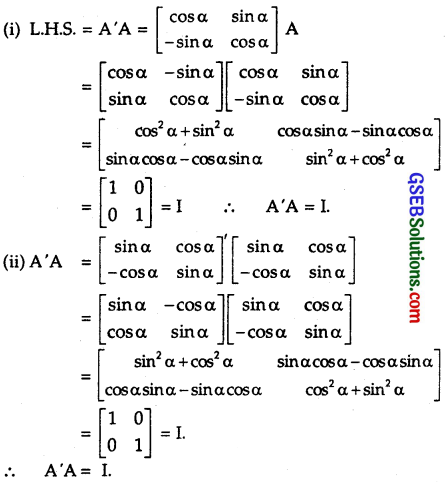Question 7.
(i) Show that the matrix A = $$\left[\begin{array}{ccc} 1 & -1 & 5 \\ -1 & 2 & 1 \\ 5 & 1 & 3 \end{array}\right]$$ is a symmetric matrix.
(ii) Show that the matrix A = $$\left[\begin{array}{ccc} 0 & 1 & -1 \\ -1 & 0 & 1 \\ 1 & -1 & 0 \end{array}\right]$$ is a skew – symmetric matrix.
Solution:
(i) For a symmetric matrix a = aij.
Now, A = $$\left[\begin{array}{ccc} 1 & -1 & 5 \\ -1 & 2 & 1 \\ 5 & 1 & 3 \end{array}\right]$$
a21 = – 1 = a12, a31 = 5 = a13
a32 = 1 = a23 a11, a22, a33 are 1, 2, 3 respectively.
Hence, aij = aji
∴ A is a symmetric matrix.
Alternatively:
A’ = $$\left[\begin{array}{ccc} 1 & -1 & 5 \\ -1 & 2 & 1 \\ 5 & 1 & 3 \end{array}\right]$$‘ = $$\left[\begin{array}{ccc} 1 & -1 & 5 \\ -1 & 2 & 1 \\ 5 & 1 & 3 \end{array}\right]$$ = A.
∴ A’ = A ⇒ A is a symmetric matrix.

(ii) For a skew symmetric matrix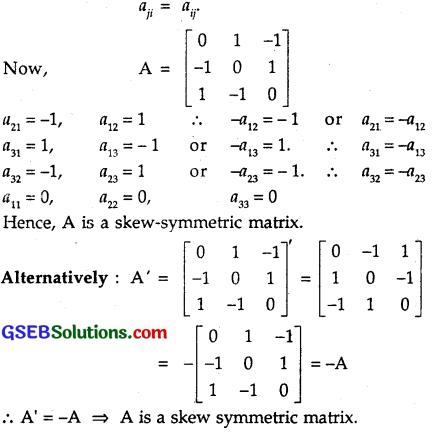Question 8.
For a matrix A = $$\left[\begin{array}{ll} 1 & 5 \\ 6 & 7 \end{array}\right]$$, verify that
(i) (A + A) is a symmetric matrix.
(ii) (A – A) is a skew symmetric matrix.
Solution:
(i) A = $$\left[\begin{array}{ll} 1 & 5 \\ 6 & 7 \end{array}\right]$$ ∴ A’ = $$\left[\begin{array}{ll} 1 & 5 \\ 6 & 7 \end{array}\right]$$
∴ A + A’ = $$\left[\begin{array}{ll} 1+1 & 5+6 \\ 6+5 & 7+7 \end{array}\right]$$ = $$\left[\begin{array}{ll} 2 & 11 \\ 11 & 14 \end{array}\right]$$
Here, a21 = 11 = a12
∴ A + A’ is a symmetric matrix.

(i) A – A’ = $$\left[\begin{array}{ll} 1 & 5 \\ 6 & 7 \end{array}\right]$$ – $$\left[\begin{array}{ll} 1 & 6 \\ 5 & 7 \end{array}\right]$$
= $$\left[\begin{array}{ll} 1-1 & 5-6 \\ 6-5 & 7-7 \end{array}\right]$$ = $$\left[\begin{array}{ll} 0 & -1 \\ 1 & 0 \end{array}\right]$$.
Here, a21 = 1, a12 = – 1 or – a12 = 1.
∴ a21 = – a12 a11 = a22 = 0
∴ A – A’ is a symmetric matrix.

Question 9.
Find $$\frac { 1 }{ 2 }$$(A +A) and $$\frac { 1 }{ 2 }$$(A-A), when A = $$\left[\begin{array}{ccc} 0 & a & b \\ -a & 0 & c \\ -b & -c & 0 \end{array}\right]$$.
Solution: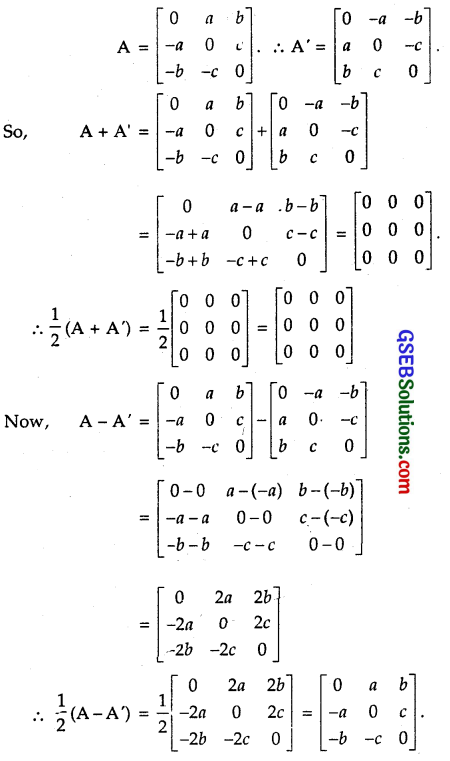Question 10.
Express the following matrices as the sum of a symmetric and a skew symmetric matrix.
(i) $$\left[\begin{array}{ll} 3 & 5 \\ 1 & -1 \end{array}\right]$$
(ii) $$\left[\begin{array}{ccc} 6 & -2 & 2 \\ -2 & 3 & -1 \\ 2 & -1 & 3 \end{array}\right]$$
(iii) $$\left[\begin{array}{ccc} 3 & 3 & -1 \\ -2 & -2 & 1 \\ -4 & -5 & 2 \end{array}\right]$$
(iv) $$\left[\begin{array}{ll} 1 & 5 \\ -1 & 2 \end{array}\right]$$
Solution: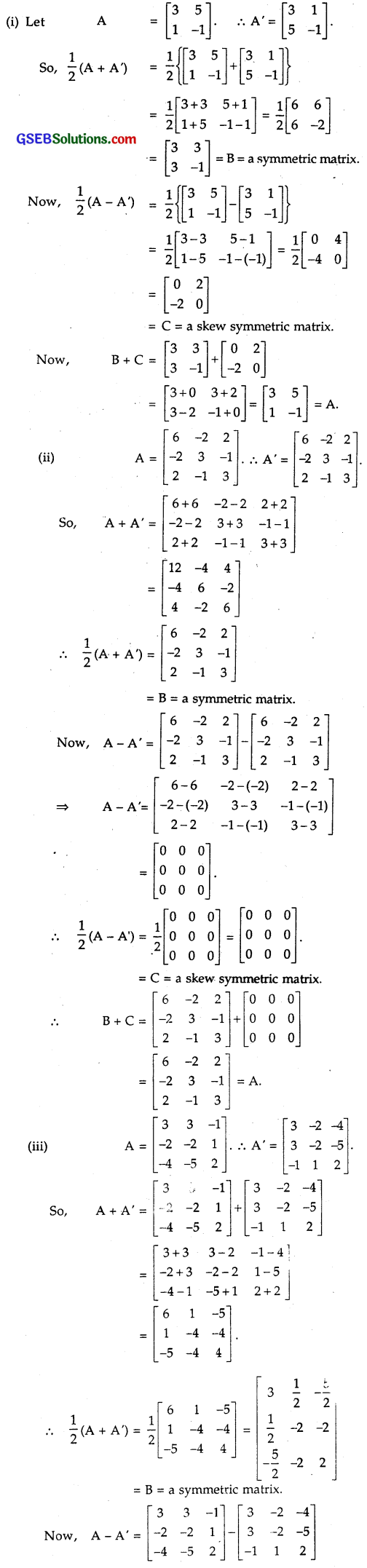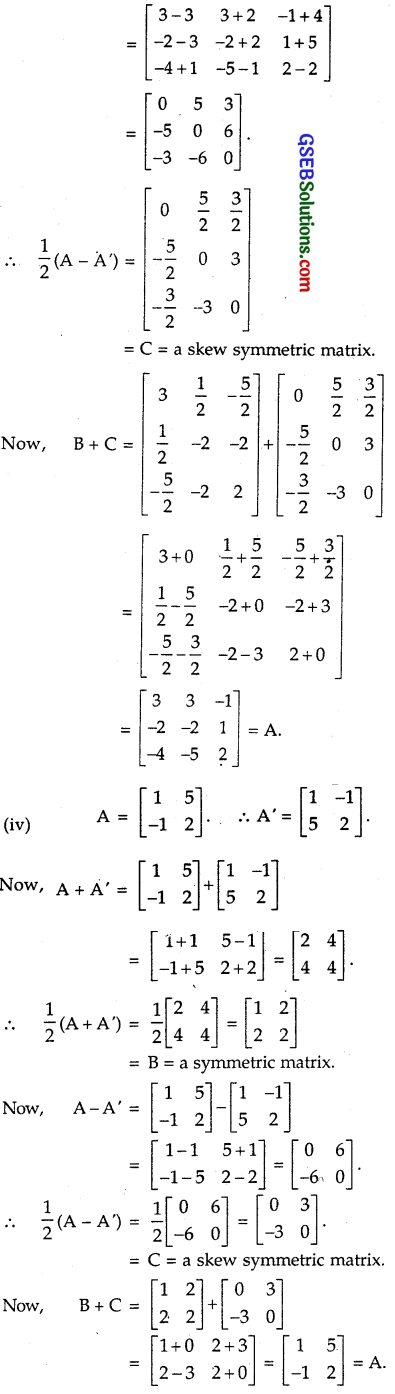Choose the correct answer in the following questions:

Question 11.
If A and B are symmetric matrices of the same order, then AB – BA is a _____.
(A) Skew symmetric matrix
(B) Symmetric matrix
(C) Zero matrix
(D) Identity matrix
Solution:
A and B are symmetric matrices.
So, A’ = A and B’ = B.
Now, (AB – BA)’ = (AB)’ – (BA)’ = B’A’ – A’B’
= BA – AB [∵ B’ = B, A’ = A]
⇒ AB – BA is a skew symmetric matrix.
∴ Part (A) is the correct answer.Question 12.
If A = $$\left[\begin{array}{cc} \cos \alpha & -\sin \alpha \\ \sin \alpha & \cos \alpha \end{array}\right]$$ then A + A’= I, if the value of α is
(A) $$\frac { π }{ 2 }$$
(B) $$\frac { π }{ 3 }$$
(C) π
(D) $$\frac { 3π }{ 2 }$$
Solution:
A = $$\left[\begin{array}{cc} \cos \alpha & -\sin \alpha \\ \sin \alpha & \cos \alpha \end{array}\right]$$ ⇒ A’ = $$\left[\begin{array}{cc} \cos \alpha & \sin \alpha \\ -\sin \alpha & \cos \alpha \end{array}\right]$$
∴ A + A’ = $$\left[\begin{array}{cc} \cos \alpha+\cos \alpha & -\sin \alpha+\sin \alpha \\ \sin \alpha-\sin \alpha & \cos \alpha+\cos \alpha \end{array}\right]$$
= $$\left[\begin{array}{cc} 2 \cos \alpha & 0 \\ 0 & 2 \cos \alpha \end{array}\right]$$ = $$\left[\begin{array}{ll} 1 & 0 \\ 0 & 1 \end{array}\right]$$
2 cos α = 1 ⇒ cos α = $$\frac { 1 }{ 2 }$$
α = $$\frac { π }{ 3 }$$
∴ Part (B) is the correct answer.